Impulse commutation is also called voltage commutation, auxiliary commutation, parallel capacitor commutation, or Class-D commutation. It is used in dc choppers and inverters. A circuit diagram of the impulse commutation of the thyristor is given in Fig. 1. Initially, T1 and TA OFF while C is charged to VS.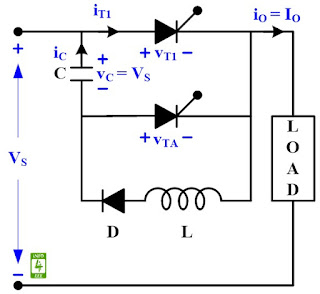Fig. 1 Circuit Diagram for impulse commutation

Case-I: At t = 0; T1 is turned ON, VS is applied across the load, io remains constant, another oscillatory circuit consisting of C, T1, L, and D is formed. iC = VS✖√(C/L)•sinωot = IP•sinωot.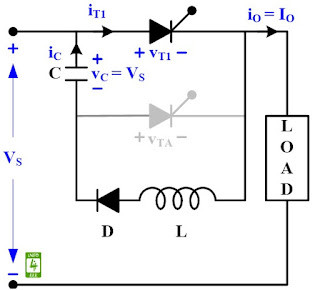Fig. 2. Equivalent circuit diagram during case-I

Case-II: At t = π/ωo, iC = 0, iT1 = io, and vC = -VS.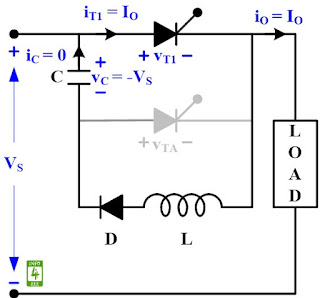Fig. 3. Equivalent circuit diagram during case-II

Case-III: At t = t1, TA is turned ON, vc applies a reverse voltage across T1, Tis turned OFF and iT1 = 0. load current flow through C and TA.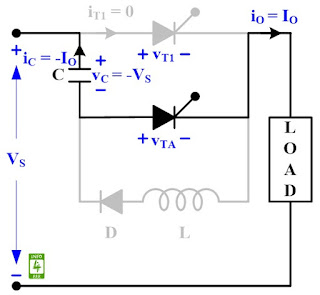Fig. 4. Equivalent circuit diagram during case-III

Case-IV: At t = t2, vc = VS, ic = 0, TA is turned OFF, and tc is called circuit turn OFF time.Fig. 5. Equivalent circuit diagram during case-IV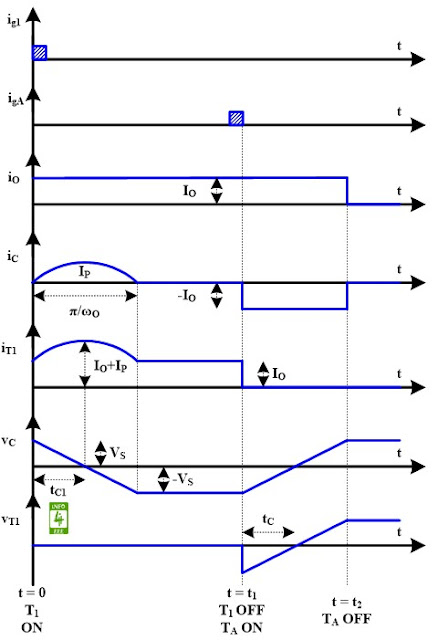Fig. 6. The waveform of impulse commutation of a thyristor.

#### Reference

Dr. P. S. Bimbhra, "Power Electronics", Khanna Publishers, Fifth Edition, 2013.

#### Author

authored 77 Technical Articles for INFO4EEE Website since 2012.